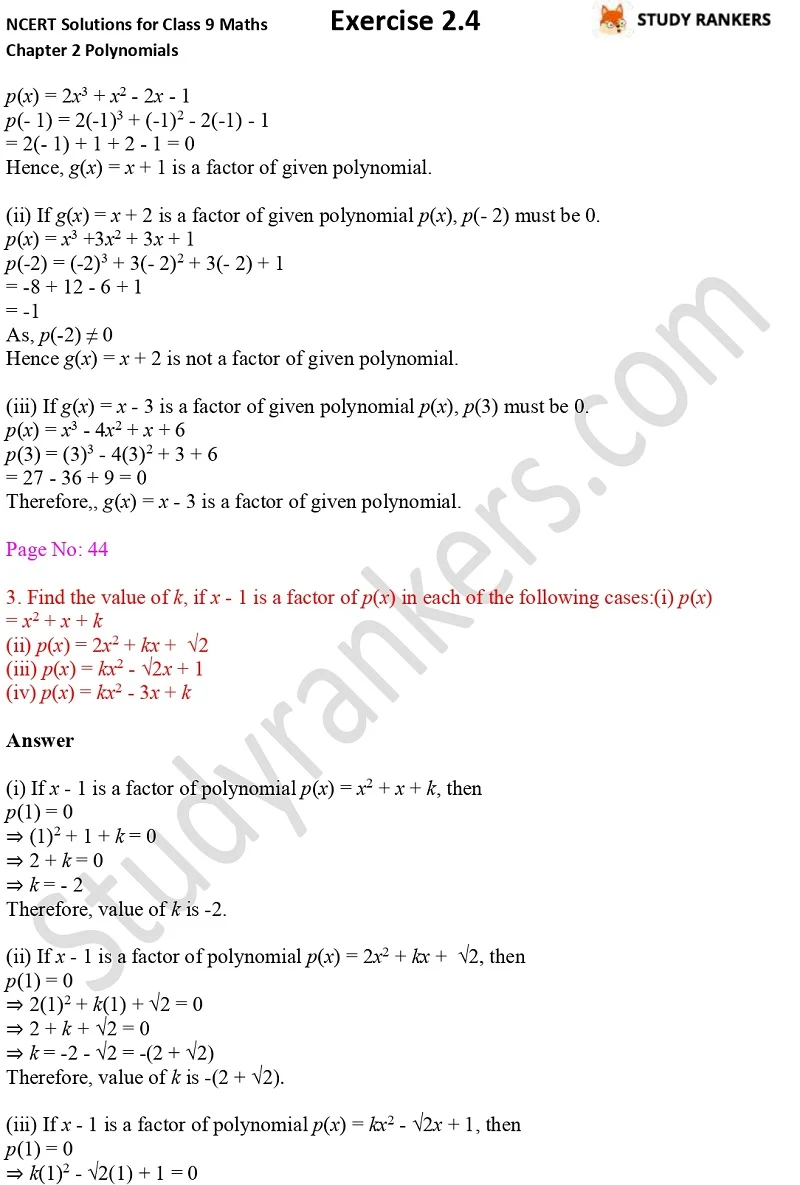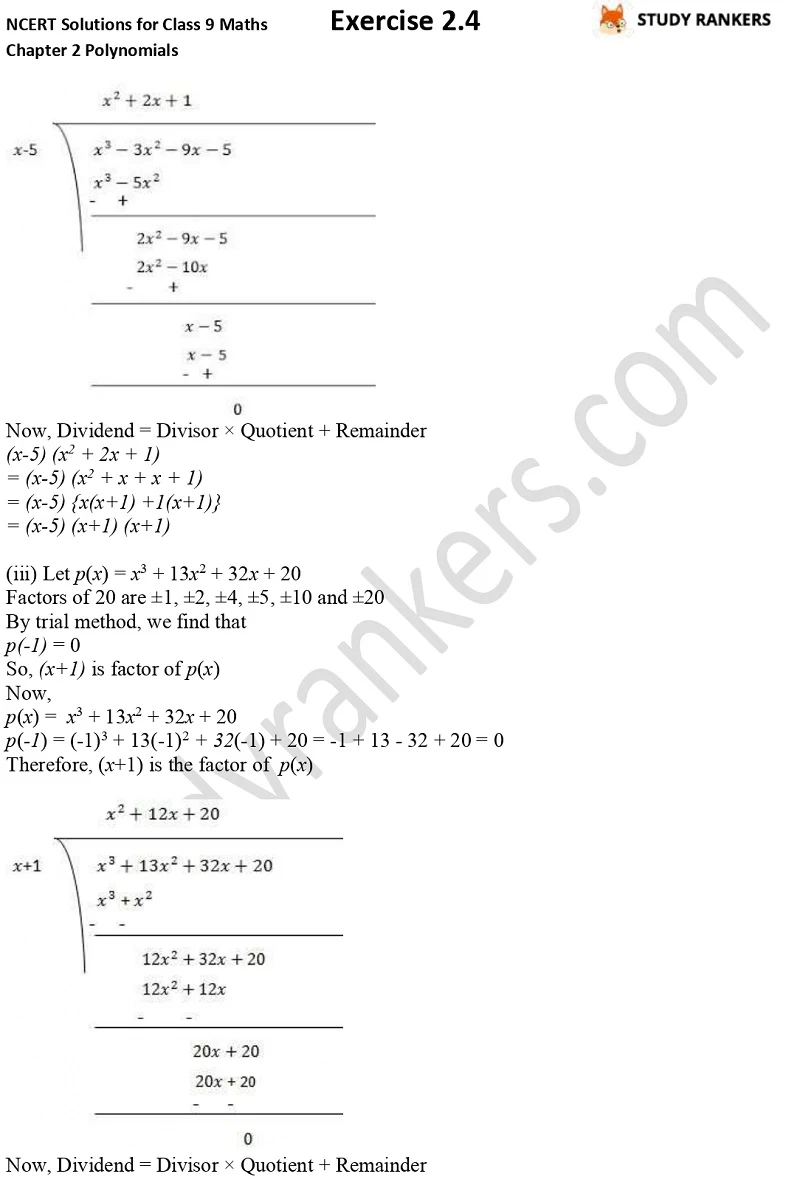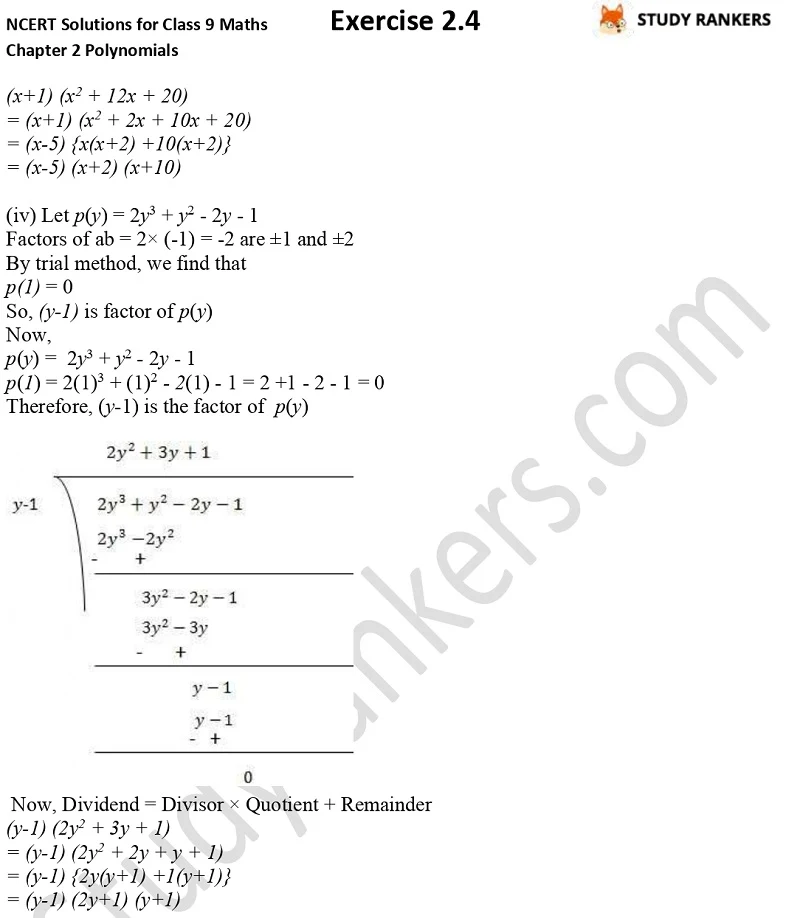## NCERT Solutions for Class 9 Maths Chapter 2 Polynomials Exercise 2.4

Chapter 2 Polynomials Exercise 2.4 Class 9 Maths NCERT Solutions are very useful in knowing the basics of the chapter and application of formulas. Here, we have provide NCERT Solutions for Class 9 Maths that are accurate and detailed prepare by Stduyrankers subject matter expert so you can excel in your exams. You can complete your homework on time and also curate your answers by taking help from these NCERT Solutions.

Exercise 2.4 has total three questions which is about find the factor using factor theorem.